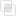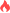python异常中else的使用

else2021-06-11 17:31:121449浏览 · 0收藏 · 0评论1、说明

2、实例

```while True:
try:
x = int(input('请输入X:'))
y = int(input('请输入Y:'))
value = x / y
print('x/y is',value)
except Exception as e:  # 发生异常时执行
print('不正确的输入：', e)
print('请重新输入')
else:  # 未发生异常时执行
break```Python实现Excel的整行插入Python中DataFrame有哪些常用方法Python中使用xlwings整行删除Python中concurrent.futures模块如何使用Python kmeans聚类的使用Python K-means算法的计算步骤Python默认值的使用注意Python特殊传参如何实现python中的两种输出语句python循环语句的两种类型python继承的多种类型python中pandas模块查看DataFramepython merge()的连接python join()合并DataFrame的操作python concat方法的作用java虚拟机中栈的运行原理846

js中自定义迭代行为的介绍718

python中del在垃圾回收的使用857